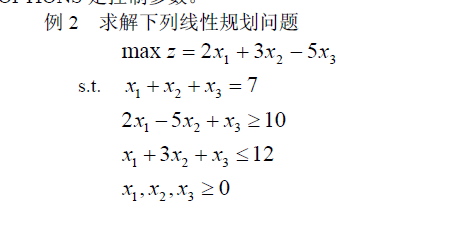# 用python做线性规划scipy.optimize.linprog(cA_ub=Noneb_ub=NoneA_eq=Noneb_eq=Nonebounds=Nonemethod='simplex'callback=Noneoptions=None)

from scipy import optimize as op
import numpy as np
c=np.array([2,3,-5])
A_ub=np.array([[-2,5,-1],[1,3,1]])
B_ub=np.array([-10,12])
A_eq=np.array([[1,1,1]])
B_eq=np.array()
x1=(0,7)
x2=(0,7)
x3=(0,7)
res=op.linprog(-c,A_ub,B_ub,A_eq,B_eq,bounds=(x1,x2,x3))
print(res)

     fun: -14.571428571428571
message: 'Optimization terminated successfully.'
nit: 2
slack: array([3.85714286, 0.57142857, 6.42857143, 7.        , 0.        ])
status: 0
success: True
x: array([6.42857143, 0.57142857, 0.        ])

09-1804-09
03-128762
09-092171
08-152万+
04-234051
05-171万+
04-154376
01-29943
07-196798
01-20163
06-021384
03-14505
05-22148
05-1614
12-23275
©️2020 CSDN 皮肤主题: 大白 设计师:CSDN官方博客